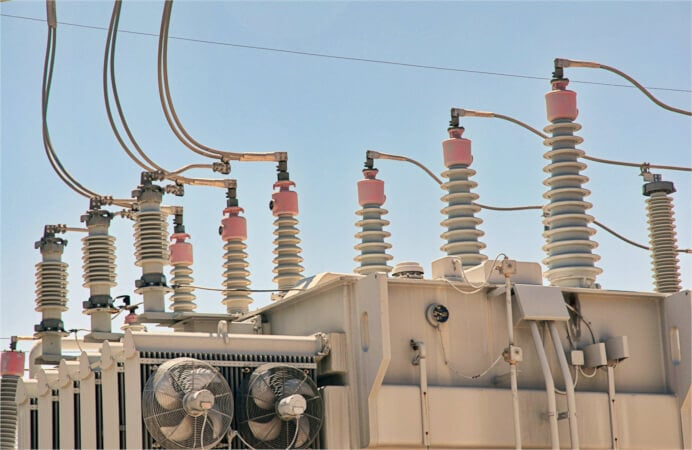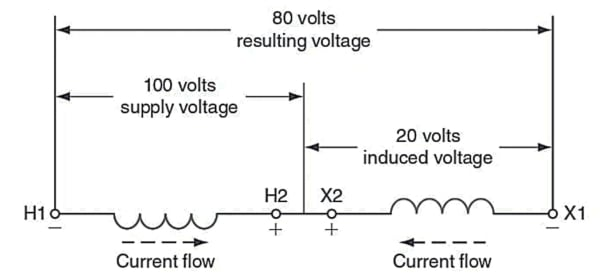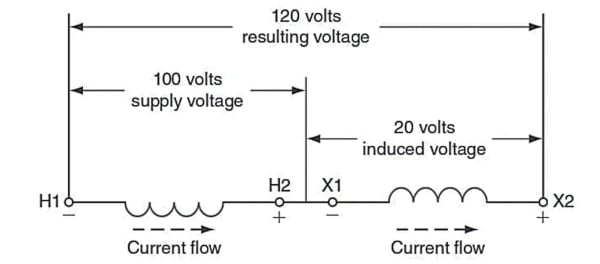Technical Article

October 03, 2023 by Ahmed Sheikh

This article covers the process of distinguishing transformer leads in cases where identifying numbers may have become unreadable over time. It discusses the challenges with small single-phase transformers and provides insights into using resistance and winding turns to discern the H and X leads accurately. The article also explains how to perform additive and subtractive polarity tests, which are crucial for determining the lead numbers and adhering to ANSI standards.### Determining if Unknown Heads Are H or X

How can you determine which one of the H leads is the H1 lead or which of the X leads is the X1 lead? If we apply power to the H windings, we will induce a voltage in the X windings. If we connect the H and X windings together, we can read a voltage across the combination of the two windings. Connecting the H winding with the applied voltage in series with the X winding with its induced voltage will result in one of two possible outcomes:

1. The X-induced voltage will add to the H-applied voltage—it will be additive.

2. The X-induced voltage will subtract from the H-applied voltage—it will be subtractive.### Subtractive Polarity Test Connection

Let’s look at Figure 1, which shows the connection we have described, to see how this can happen.

The positive ends of both of the windings are connected together. This is like connecting the positive ends of two batteries together. Like charges repel, and the current flow in one winding is opposite to the current flow in the other winding; therefore, the resulting voltage is less than the applied voltage. The induced voltage in the X winding opposes the supply voltage. In the connection above, if the H winding had a voltage of 100 V applied to it and the X winding had a voltage of 20 V induced in it, the resulting voltage would be 80 V. The 20 V induced in the X winding is in opposition to the 100 V applied to the H winding, so the net voltage is the difference between the two 80 V. This is a subtractive relationship between the H and X windings.

In Figure 2, both transformer windings are still connected in series, and power is applied to the H winding as before. The two windings are now connected, so the positive end of the H winding is connected to the negative end of the X winding. This is like connecting the negative end of one battery to the positive end of another battery. Unlike charges attract, and the current flow in both windings is in the same direction; therefore, the voltages of both windings add together, and the resulting voltage is greater than the applied voltage. This is an additive relationship between the H and X windings. Connecting the H and X windings in series, supplying power to the H winding, and measuring the resulting voltage as we have described is called performing a polarity test on the windings.### Determining H and X Lead Numbers

We now can determine which of the transformer leads should be labeled with a 1. Because the transformer is connected to an AC voltage, each end of the transformer H winding will be 1 at a different point in time, so it does not matter which end of the H winding we label with a 1. Once we label one end of the H winding with a 1, however, it does matter which end of the X winding is labeled with a 1. Remember that ANSI standards require that the H1 and the X1 leads have the same polarity at any instant in time because they are both labeled with an odd number. The lead of the X winding that has the same polarity as the H1 lead must also be labeled with a 1.

We can determine the polarity of the X lead in relation to the H1 lead by performing a polarity test—that is, connecting the two windings in series as we have just described and measuring the resulting voltage.

If the voltage is less than the applied voltage, then the X winding lead that is connected to the H winding lead must have the same polarity as the H winding lead and, as a result, the same number—even or odd—as the H winding lead. The leads of the two windings that are connected together must both have even, or odd numbers attached to them to comply with ANSI standards.

If the resulting voltage is more than the applied voltage, then the X winding lead that is connected to the H winding lead must have the opposite polarity of the H winding lead and, as a result, the opposite number as the H winding lead. The leads of the two windings that are connected together must have different numbers—even or odd—attached to them to comply with ANSI standards.

In short, if the windings are in a subtractive relationship, the lead numbers will be the same. If the windings are in an additive relationship, the lead numbers will be opposite.